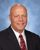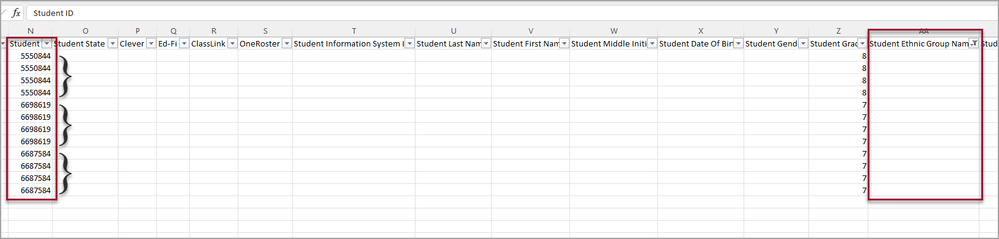cancel
Showing results for
Did you mean:Helper IV

## Using countblank in one column and get a distinct count of another column values

I created the countblank measure below, but I ultimately would like a distinct count on the number of students who are missing a value in the Student Ethnic Group Name field.

Missing Ethnicity = COUNTBLANK('86362'[Student Ethnic Group Name])

I was thinking that if the Student ID field was used for the distinct count (3) that would work, instead of the 12 I get for a result with only COUNTBLANK,2 ACCEPTED SOLUTIONSSolution Sage

You can try the following measure that takes a distinct count of student IDs when the ethnic group name is blank.

Missing Ethnicity =
CALCULATE(
DISTINCTCOUNT('86362'[Student ID]),
OR(ISBLANK('86362'[Student Ethnic Group Name]), '86362'[Student Ethnic Group Name] = "")
)Solution Sage

Yep.

Amend the measure to

Missing Ethnicity =
var _calc =
CALCULATE(
DISTINCTCOUNT('86362'[Student ID]),
OR(ISBLANK('86362'[Student Ethnic Group Name]), '86362'[Student Ethnic Group Name] = "")
)
Return
IF(
ISBLANK(_calc),
0,
_calc
)
6 REPLIES 6Helper IV

@jgeddes I'm using a Card to display the results.Helper IV

@jgeddes is it possible to add to the formula to get a "0" (zero) instead of (Blank)? when there are no students missing ethnicity?Solution Sage

Yep.

Amend the measure to

Missing Ethnicity =
var _calc =
CALCULATE(
DISTINCTCOUNT('86362'[Student ID]),
OR(ISBLANK('86362'[Student Ethnic Group Name]), '86362'[Student Ethnic Group Name] = "")
)
Return
IF(
ISBLANK(_calc),
0,
_calc
)Helper IV

@jgeddes thanks for the addition to get the zero result. Take care!Helper IV

@jgeddes that worked. Thank you so much!Solution Sage

You can try the following measure that takes a distinct count of student IDs when the ethnic group name is blank.

Missing Ethnicity =
CALCULATE(
DISTINCTCOUNT('86362'[Student ID]),
OR(ISBLANK('86362'[Student Ethnic Group Name]), '86362'[Student Ethnic Group Name] = "")
)